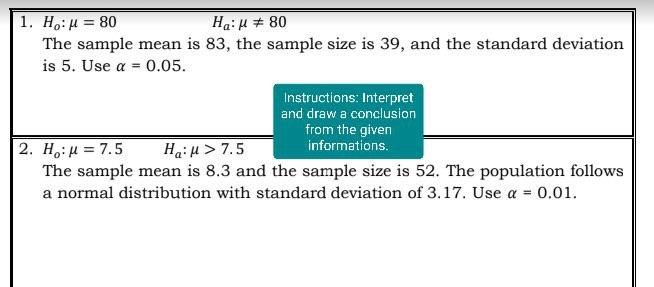# Question Please answer the questions on the picture. Thank You! 1. Ho: μ = 80 Ha:μ # 80 The sample mean is 83, the sample size is 39, and the standard deviation is 5. Use a = 0.05. Instructions: Interpret and draw a conclusion from the given informations. 2. Ho: μ = 7.5 Ha: μ > 7.5 The sample mean is 8.3 and the sample size is 52. The population follows a normal distribution with standard deviation of 3.17. Use a = 0.01.WS7KEH The Asker · Probability and Statistics